# How many hg of oxygen are in 9.999 pg of cobaltous phosphite nanohydrate?

How many hg of oxygen are in 9.999 pg of cobaltous phosphite nanohydrate?

We need at least 10 more requests to produce the answer.

0 / 10 have requested this problem solution

The more requests, the faster the answer.

All students who have requested the answer will be notified once they are available.

#### Earn Coin

Coins can be redeemed for fabulous gifts.

Similar Homework Help Questions
• ### 41. Suppose you have half a mole of sulfur atoms. How many sulfur atoms do you...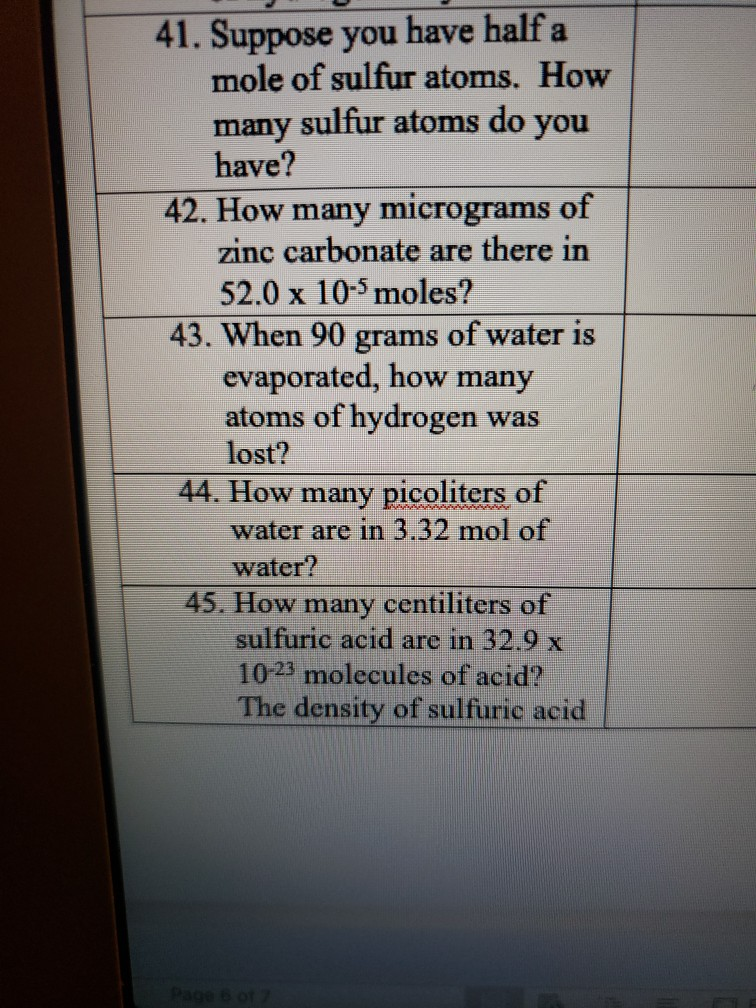41. Suppose you have half a mole of sulfur atoms. How many sulfur atoms do you have? 42. How many micrograms of zinc carbonate are there in 52.0 x 10-5 moles? 43. When 90 grams of water is evaporated, how many atoms of hydrogen was lost? 44. How many picoliters of water are in 3.32 mol of water? 45. How many centiliters of sulfuric acid are in 32.9 x 10-23 molecules of acid? The density of sulfuric acid Page 6...

• ### How many moles of oxygen will occupy 728 ml at 760 mm of Hg and 25...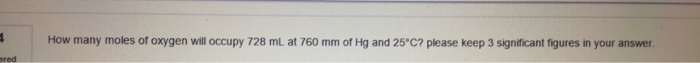How many moles of oxygen will occupy 728 ml at 760 mm of Hg and 25 degree C? please keep 3 significant figures in your answer

• ### Acetylene (c2h2) burns with oxygen to form carbon dioxide and water . How many liters of...

Acetylene (c2h2) burns with oxygen to form carbon dioxide and water . How many liters of oxygen at 45degres and 650 mm hg are needed to burn 25.0 l of acetyleneat 37 degrees and 820 mm hg ?

• ### the composition of individuals in KCIO SCH- 90- +- How many gram of Oxygen is in...the composition of individuals in KCIO SCH- 90- +- How many gram of Oxygen is in 5.6 gram of Mgo 3- Find the empirical formula of a compound with the following composition, Hg 80.69% S 6.436% 0 12.87% How many atoms are in 20 gram of K3PO4?

• ### How many ng of lithium are in 83.3 pg of lithium nitrate pentahydrate?

How many ng of lithium are in 83.3 pg of lithium nitrate pentahydrate?

• ### If the atmosphere contains 21% oxygen at 730 mm Hg, then the partial pressure of oxygen...

If the atmosphere contains 21% oxygen at 730 mm Hg, then the partial pressure of oxygen would be:

• ### How many gram of Oxygen is in 5.6 gram of Mg(OH)2. 5- Find the empirical formula of a compound with the following c...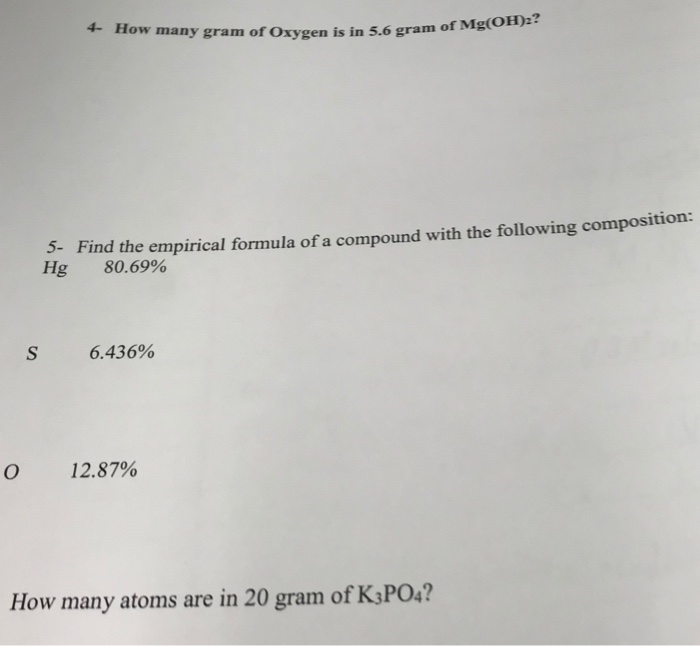How many gram of Oxygen is in 5.6 gram of Mg(OH)2. 5- Find the empirical formula of a compound with the following composition: Hg 80.69% 6.436% 0 12.87% How many atoms are in 20 gram of K3PO4?

• ### 6) Liquid mercury (p = 13.56 g Hg/mL Hg) reacts with oxygen gas to form a...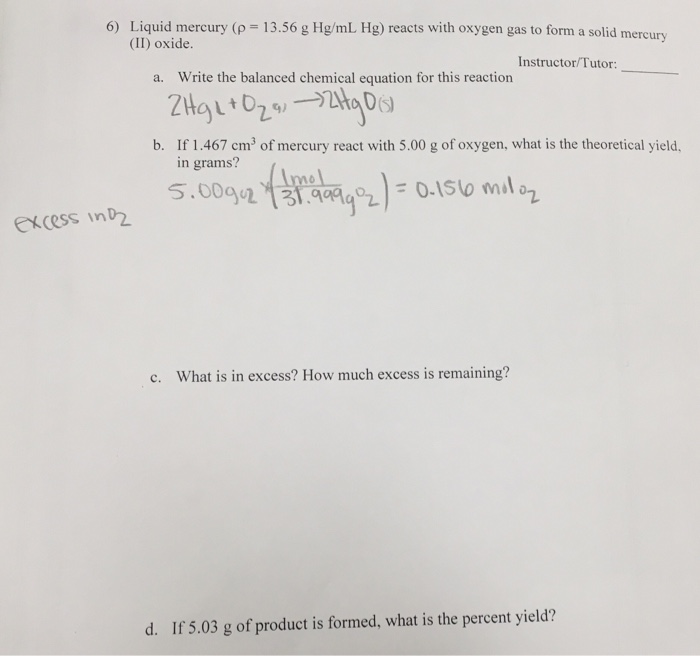6) Liquid mercury (p = 13.56 g Hg/mL Hg) reacts with oxygen gas to form a solid mercury (II) oxide. Instructor/Tutor: a. Write the balanced chemical equation for this reaction ZHg2+O29, ~2Hg Ois) of mercury react with 5.00 g of oxygen, what is the theoretical yield, b. If 1.467 cm in grams? 5.00gu2 na 1992) = 0.1510 molaz excess in D c. What is in excess? How much excess is remaining? d. If 5.03 g of product is formed, what...

• ### in a gas with a total pressure of 600mm Hg that contains 10% oxygen 20% carbon...

in a gas with a total pressure of 600mm Hg that contains 10% oxygen 20% carbon dioxide and 70% nitrogen the partial pressure of oxygen would be how much mmHg

• ### How many oxygen atoms are there in 95g of barium chromate? How many oxygen atoms are there in 95 g of barium chro...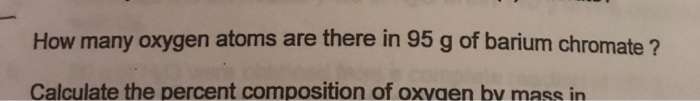How many oxygen atoms are there in 95g of barium chromate? How many oxygen atoms are there in 95 g of barium chromate? Calculate the percent composition of oxygen by mass in